#### Theory of Dynamic Characteristics III and Inverter Fall Time

Inverter Fall Time:

The equation obtained explaining the collector current as a function of time is as follows: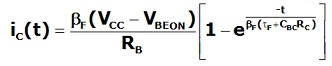But, as VO = VCC - iC RC, then during the drop of the output voltage of inverter, we have: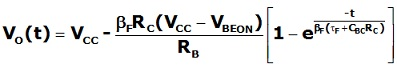This gives the impression that output voltage is heading from VCC towards a big, negative voltage, however, VCC – σ (VCC - VBEON) as can be seen in figure below: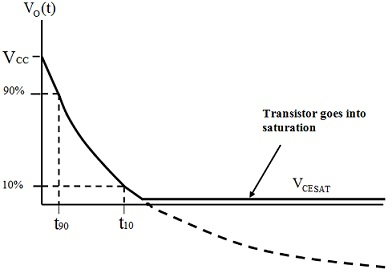Figure: Output Voltage of Transistor Inverter for Transistor Turn-On

Though, the transistor reaches saturation when iC = ICMAX. At this point, the output voltage reaches its least value VCESAT.

If VCC >> VBE ON then VCC - VBE ON ≈ VCC and as (βF RC)/RB = σu then the expression for Vo(t) can be as follows: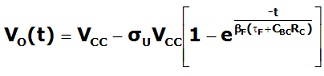To get the fall-time for inverter, the times at which the output voltage reaches 90% and 10% correspondingly of the supply voltage should be determined.

Then, at t = t90, Vo(t) = 0.9VCC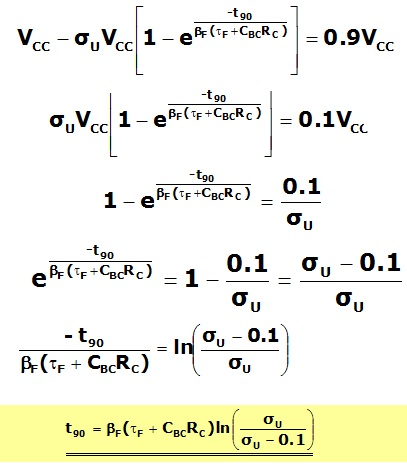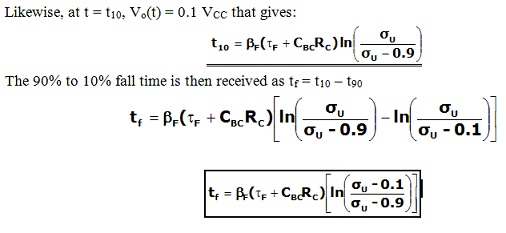Usually, βF =50, RC =1kΩ, CBC = 0.5pF and τF =0.2ns that gives:

CBC RC = 0.5 x 10-12 x 103 = 0.5 ns whereas τF = 0.2ns

Then,

βFF + CBC RC) = 50 (0.5 + 0.2) x 10-9 = 35 ns

Note that both external load and internal properties of the transistor are uniformly significant in determining the switching speed of inverter.

When no overdrive were utilized, σu = 1then ln [(1 – 0.1)/(1 – 0.9)] =ln 9 = 2.2

However with an overdrive factor, σu = 5then, then ln [(5– 0.1)/(5 – 0.9)] =ln 1.195 = 0.18

And hence, tf = 35 x 10-9 x 0.18 = 6.3ns

Note that the base overdrive significantly decreases the switching time throughout turn-on of the transistor.

Storage Time, tS

During storage time, the transistor operates in saturation mode. For this whole period, the collector current remains at its maximum value, ICMAX and the output voltage of inverter remains low, in spite of the fact that input voltage has switched from high to low. This time is spent eliminating the surplus overdrive charge from the base region as illustrated in figure shown below.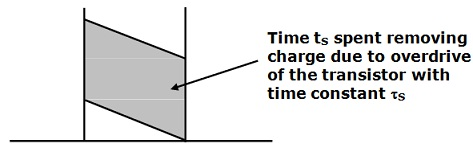Figure: Storage Time Due to Removal of Overdrive Charge

In order to model this procedure accurately, a more complete charge control model is required that takes account of charge flow in both forward and reverse directions via the transistor. This can be shown that the forward charge concentration consists of a solution of the form: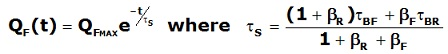Where, τs is termed to as the saturation time constant, usually 20-25ns. This can be seen thus that, while the base overdrive speeds up the transistor turn-on, it slows down the transistor turn-off.

Rise-Time, tR

During this time, the transistor again operates in forward active mode, however with the transistor turning off and collector current falling from ICMAX to zero. The similar charge control equations apply as in case of transistor turn-on. The difference is that, this time charge is being eliminated from the base and as a result, the base current is negative as shown in figure below.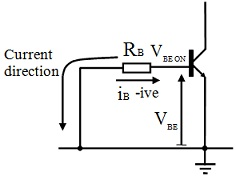Figure: Elimination of Stored Charge during Transistor Turn-Off

When the base-emitter voltage is taken as constant at VBE ON whereas in the forward active mode then iB = - (VBE ON/RB) and the charge control equations become:

iC = QF/TF – dQBC/dt

iB = QF/TBF + dQF/dt + dQBC/dt = - (VBE ON/RB)

The similar substitutions apply as in case of the fall-time analysis and hence the equation for base current, that has to be solved to get an expression for collector current, is as follows: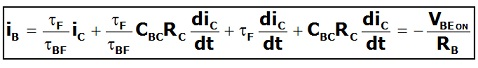Latest technology based Electrical Engineering Online Tutoring Assistance

Tutors, at the www.tutorsglobe.com, take pledge to provide full satisfaction and assurance in Electrical Engineering help via online tutoring. Students are getting 100% satisfaction by online tutors across the globe. Here you can get homework help for Electrical Engineering, project ideas and tutorials. We provide email based Electrical Engineering help. You can join us to ask queries 24x7 with live, experienced and qualified online tutors specialized in Electrical Engineering. Through Online Tutoring, you would be able to complete your homework or assignments at your home. Tutors at the TutorsGlobe are committed to provide the best quality online tutoring assistance for Electrical Engineering Homework help and assignment help services. They use their experience, as they have solved thousands of the Electrical Engineering assignments, which may help you to solve your complex issues of Electrical Engineering. TutorsGlobe assure for the best quality compliance to your homework. Compromise with quality is not in our dictionary. If we feel that we are not able to provide the homework help as per the deadline or given instruction by the student, we refund the money of the student without any delay.